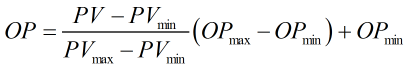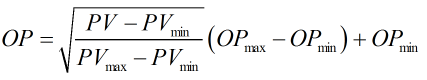# C245 – DAC Output Check

## Description

This calculation performs an DAC check which can be used for the calibration of equipment where there is a linear relationship between the input and the output.

Kelton calculation reference C245

KIMS calculation reference K123

## Options

### Primary variable

• Scalar
• Factor
• Pressure
• Temperature
• Volume flow rate
• Mass flow rate

This option allows user to select the relevant input type.

### Transmitter Output

• Current
• Voltage

This option allows user to select whether the transmitter output is a current or a voltage.

### Display output as voltage

Converts output current into a voltage.

### Display output as current

Converts output voltage into a current.

### Square root extraction

This option is selected if the input has a square root relationship with the output.

## Calculation

### Output Value

The output value is calculated by:Or when square root extraction is applied:Where PV = Primary variable value PVmax = Primary variable maximum value PVmin = Primary variable minimum input value OPmax = Upper range output value (which corresponds to the set maximum input value) for the instrument being calibrated OPmin = Lower range output value (which corresponds to the set minimum input value) for the instrument being calibrated

Back